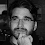## Tuesday, March 11, 2014

### Migrating from Moq to NSubstituteMocking is a necessary evil for unit testing.

Fortunately frameworks like NSubstitute make it painless setup your mock services. NSubstitute offers a fluent API that requires few lambdas and no calls to an Object property. You just get back the interface that you are substituting and work with it directly. Frankly, NSubstitute is so easy to work with that it almost seems like magic!

Below is a visual representation of equivalent commands between Moq and NSubstitute:

### NSubstitute

`[Fact]`
`public void NSubstitute()`
`{`
`    var tester = Substitute.For<ITester>();`
` `
`--------------------------------------------`
`            `
`    var voidArg = String.Empty;`
` `
`    tester`
`        .When(t => t.Void(Arg.Any<string>()))`
`        .Do(i => voidArg = i.Arg<string>());`
` `
`    tester.Void("A");`
`    Assert.Equal("A", voidArg);`
` `
`--------------------------------------------`
` `
`    tester`
`        .Bool()`
`        .Returns(true);`
`                `
`    var boolResult = tester.Bool();`
`    Assert.Equal(true, boolResult);`
` `
`--------------------------------------------`
` `
`    tester`
`        .Int`
`        .Returns(1);`
` `
`    var intResult = tester.Int;`
`    Assert.Equal(1, intResult);`
` `
`--------------------------------------------`
` `
`    tester.Received(1).Void("A");`
` `
`--------------------------------------------`
` `
`    tester.DidNotReceive().Void("B");`
`}`

### Moq

`[Fact]`
`public void Moq()`
`{`
`    var tester = new Mock<ITester>();`
`            `
`    // Setup a callback for a void method. -------`
` `
`    var voidArg = String.Empty;`
` `
`    tester`
`        .Setup(t => t.Void(It.IsAny<string>()))`
`        .Callback<string>(s => voidArg = s);`
` `
`    tester.Object.Void("A");`
`    Assert.Equal("A", voidArg);`
` `
`    // Setup the result of a method. -------------`
` `
`    tester`
`        .Setup(t => t.Bool())`
`        .Returns(true);`
` `
`    var boolResult = tester.Object.Bool();`
`    Assert.Equal(true, boolResult);`
` `
`    // Setup the result of a property. -----------`
` `
`    tester`
`        .SetupGet(t => t.Int)`
`        .Returns(1);`
` `
`    var intResult = tester.Object.Int;`
`    Assert.Equal(1, intResult);`
` `
`    // Ensure that a function was called. --------`
` `
`    tester.Verify(m => m.Void("A"), Times.Once);`
` `
`    // Ensure that a function was NOT called. ----`
` `
`    tester.Verify(m => m.Void("B"), Times.Never);`
`}`

Enjoy,
Tom

1.You're just doing Moq and mocking itself wrong.

// Ensure that a function was called. --------

testerMock.Verify(m => m.Void("A"), Times.AtLeastOnce());

// Ensure that a function was NOT called. ----

testerMock.Verify(m => m.Void("B"), Times.Never());

1.I have updated the sample code.
Thanks for pointing that out!

2.For NSub, you can also do `tester.DidNotReceive().Void("B")`.

The command mapping really shows NSub owes a lot to Moq. :)

3.Updated! Thanks for the input.
David, keep up the great work!

4.don't know what it was earlier, but corrected code still not as pretty as nsub's: lambda is still here. so i assume you just misusing word "just" in your comment =)) it should be ridden of, as code was ridden of lambdas in nsub =)

2.thanks..I was thinking about which of them to learn and use.NSub seems better.